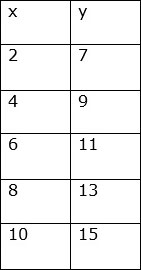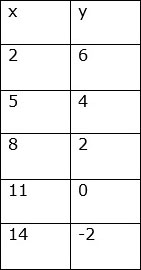# Increasing or Decreasing Pattern from a Table of Values

#### Excel add-in with c# VSTO and web

7 Lectures 1.5 hours

#### Word add-in using c# vsto and web types

8 Lectures 1 hours

#### The Ultimate Guide To Add Social Media Login to PHP Web Apps

17 Lectures 2 hours

Consider a table of values; represented by an independent variable (x) and a dependent variable (y). We often use a table of values to display the values we record. Using the table, we can find the relationship between the two variables and write it as a rule. There may be an increasing or decreasing pattern in the relationship between the two variables.

Example

Consider that the total cost (y) of x items is two times the no of items, i.e., y =2 x. The total cost (y) increases as the number of items (x) increase. So it is clear that there is an increasing pattern here in the values of the variables.

Consider the values of the variables in the table given below.### Solution

Step 1:

Consider pairs of consecutive x values and find the difference.

4 – 2 = 6 – 4 = 8 – 6 = 10 – 8 = 2

Step 2:

Similarly consider pairs of consecutive y values and see the difference.

9 – 7 = 11 – 9 = 13 – 11 = 15 – 13 = 2

Step 3:

We find that for 2-unit increase in x, there is a 2-unit increase in the y value. So we see an increasing pattern in this table of values.

Consider the values of the variables in the table given below.### Solution

Step 1:

Consider pairs of consecutive x values and find the difference.

5 – 2 = 8 – 5 = 11 – 8 = 14 – 11= 3

Step 2:

Similarly consider pairs of consecutive y values and see the difference.

4 – 6 = 2 – 4 = 0 – 2 = -2 – 0 = - 2

Step 3:

We find that for 3-unit increase in x, there is a 2-unit decrease in the y value. So we see a decreasing pattern in this table of values.